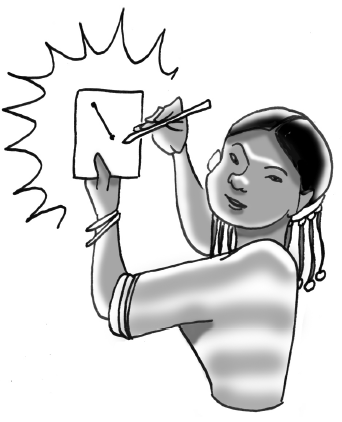### Home > CCA > Chapter 3 > Lesson 3.2.2 > Problem3-51

3-51.Jamila wants to play a game called “Guess My Line.” She gives you the following hints: “Two points on my line are $(1,1)$ and $(2,4)$."

1. What is the slope of her line? A graph of the line may help.

2. What is the $y$-intercept of her line?

$y$-intercept $=(0,−2)$

3. What is the equation of her line?

Use the rate of growth and the $y$-intercept to write an equation for the line. $y=mx+b$

Use the eTool below to complete the graph of the line and help solve each part.
Click the link at right for the full version of the eTool: 3-51 HW eTool